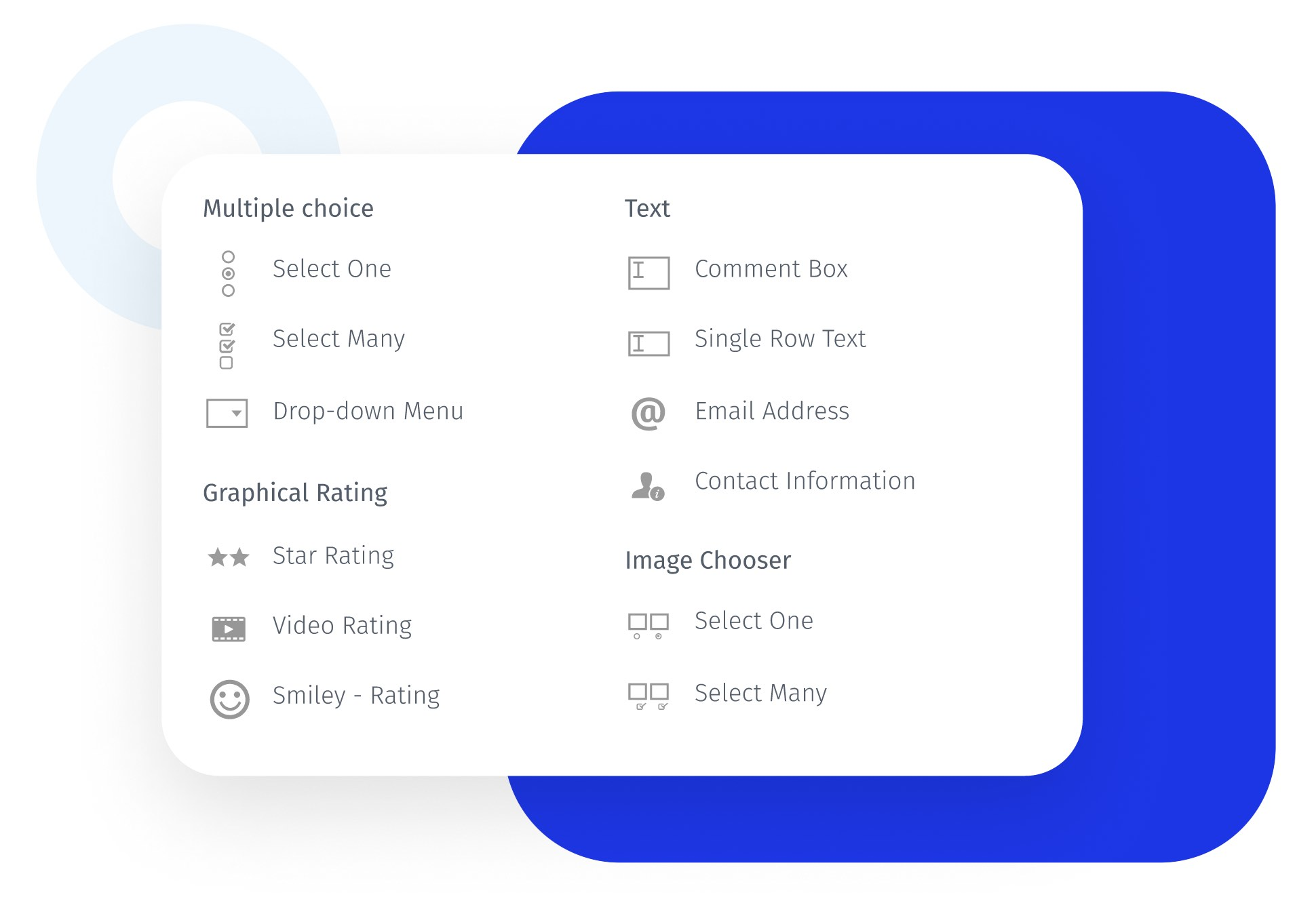# Constant sum question#### What is a constant sum question?

A constant sum question is used in market research surveys in which respondents can allocate the number of points to the specified criteria or options.

The total of these points can be defined by the survey creator while designing the survey, which will be the maximum points available to the respondents while answering this question. Constant sum question type gives an understanding of how respondents value each answer option. If customers feel that certain features of a product or service are more important than others, they will give more points to those features.

Constant sum scale is a great way to create differentiation among data sets. It helps you find out which factors are important and which are not for respondents. They are especially helpful in cases where many factors are critical and overlap in some sense. Other question types such as likert scale can find out what matters most to the respondents, but they can't highlight the variance factor.

## Example of a constant sum question

You can ask respondents to allocate 100 points on how they spend their income. Give them options and ask to assign points in order of importance. Say, they spend \$40 on groceries, \$20 on entertainment, \$30 on utilities and \$10 for miscellaneous expenses. Thus, you can find out groceries is the most important expense for the respondents followed by the utilities.## Uses of a constant sum question

Constant sum question can be used while calculating percentages, school assignments, finances, understanding customer choices, etc. This type of question is useful when you want to understand how respondents allocate points to each answer item. You can also find out to what extent do they give importance to the answer choices.

With rank order questions too, you can find out the order in which respondents consider the answer options as important to them. However, you cannot know the extent to which an answer option is important. With the constant sum scale in survey, you can find the relative weight given to the options.

## Advantages of using constant sum questions in surveys

A constant sum question permits the collection of ratio data, i.e., the weight of various options in the total sum of 100. You can also find out the ratio of various options and the importance they hold relative to each other.

• Easy to respond: To answer a constant sum question in survey, respondents can use the slider and it will automatically populate the points in the boxes. They don't have to type digits to answer such questions.

• Accurate survey data: Respondents allocate points to different attributes based on their choices. The constraint of total sum highlights how they assign relative importance to each option. This ensures better data accuracy.

• Easy to employ: Constant sum scale offers deep insights into customer preferences. You can find out which option is the most important to the customers and by what factor.

## How to use a constant sum question in your surveys?

Check out our constant sum question help document for a better understanding of how it works.